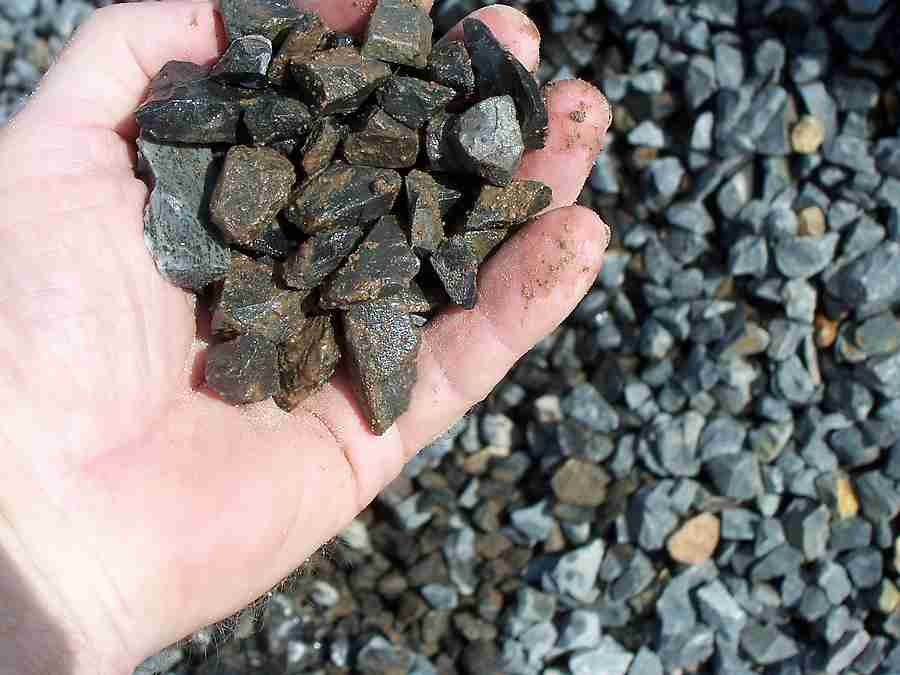# HOW TO BLEND AGGREGATE USING FINENESS MODULUS VALUE?

Sometime it may happen that, the aggregate available at site is not conforming to the desired grading. In such case two or more aggregates from different sources may be combined to get the required grading.

Fineness modulus value can be used as a technical way to blend coarse aggregate with fine aggregate to produce desired grading. Follow the steps shown below to blend aggregate using fineness modulus value.## Step by Step Guide for Blending Aggregates Using Fineness Modulus Value

### Step-1 (Calculate Fineness Modulus of Fine Aggregate)

• Sieve the fine aggregate using sieves ranging from 80 mm to 150 micron.
• Calculate the cumulative percentage of retained aggregate on each sieve.
• Add all the values of Percentage of retained on each sieve.
• Divide the sum, calculated as above, by 100. This gives the fineness modulus value of fine aggregate. Let it be F1.

### Step-2 (Calculate Fineness Modulus of Coarse Aggregate)

• Sieve the coarse aggregate using sieves ranging from 80 mm to 150 micron.
• Calculate the cumulative percentage of retained aggregate on each sieve.
• Add all the values of Percentage of retained on each sieve.
• Divide the sum, calculated as above, by 100. This gives the fineness modulus value of fine aggregate. Let it be F2.

### Step-3 (Calculate Fineness Modulus of Combined Aggregate)

• Now from the specified grading requirement, calculate fineness modulus value of combined aggregate.
• If the specified grading limit is given in percentage of passing, then subtract each of percentage of passing value from 100. This will give you the percentage of retained on each sieve.
• Add all the values of Percentage of retained on each sieve.
• Divide the sum, calculated as above, by 100. This gives the fineness modulus value of fine aggregate. Let it be F.

### Step-4 (Calculating Ratio of Fine Aggregate to Coarse Aggregate)

• Let “x” is the percentage of fine aggregate to be combined with coarse aggregate. x can be calculated using the formula given below.

x = [(F2-F)/(F-F1)]*100

Where,

F1 = Fineness modulus value of fine aggregate

F2 = Fineness modulus value of coarse aggregate

F = Fineness modulus value of combined aggregate

After finding the percentage of fine aggregate to be combined with the coarse aggregate by the above formula, their proportion can be calculated.

## Worked Out Example

### Calculating Fineness Modulus Value of Fine Aggregate (F1)

 Sieve Size (mm) Weight Retained (g) Cumulative Weight Retained (g) Cumulative percentage of weight retained (%) 80 0 0 0 40 0 0 0 20 0 0 0 10 0 0 0 4.75 10 10 2 2.36 50 60 12 1.18 50 110 22 0.600 95 205 41 0.300 175 380 76 0.150 85 465 93 < 0.150 35 500 Total 500 g Total = 246

So the fineness modulus value of fine aggregate (F1) = 246/100 = 2.46

### Calculating Fineness Modulus Value of Coarse Aggregate (F2)

 Sieve Size (mm) Weight Retained (kg) Cumulative Weight Retained (kg) Cumulative percentage of weight retained (%) 80 0 0 0 40 0 0 0 20 6 6 40 10 5 11 73.3 4.75 4 15 100 2.36 – 100 1.18 – 100 0.600 – 100 0.300 – 100 0.150 – 100 < 0.150 – 100 Total 15 kg Total = 713.3

So the fineness modulus value of coarse aggregate (F2) = 713.3/100 = 7.133

### Calculating Fineness Modulus Value of Combined Aggregate (F)

 Sieve Size (mm) Percentage of passing (%) Percentage of retained (%) 80 100 0 40 100 0 20 98 2 10 61 39 4.75 42 58 2.36 35 65 1.18 28 72 0.600 22 78 0.300 5 95 0.150 0 100 < 0.150 0 Total = 509

So the fineness modulus value of combined aggregate (F) = 509/100 = 5.09

### Calculating proportion of Fine Aggregate to Coarse Aggregate

Percentage of fine aggregate to be combined with coarse aggregate, (i.e. x) is

= [(7.133-5.09)/(5.09-2.46)]*100

= (2.04/2.63)*100

= 77%

This means that for every 100 kg of coarse aggregate, we need to blend 77 kg of fine aggregate, to get the specified grading of combined aggregate.

1.••